• 基本初等函数考点,基本初等函数考点高考例题及解析
• 函数的概念与基本初等函数考点解析,函数的概念与基本初等函数知识点总结
• 函数的概念及性质，基本初等函数的概念性质图像，初等函数
• 2019高考数学一轮复习第2章函数与基本初等函数第8课时幂函数及基本初等函数的应用练习理
• 辽宁省沈阳市2017_2018学年高中数学暑假作业集合函数基本初等函数3基本函数
• 本文介绍了基本初等函数的分类，基本初等函数的图像曲线 以及函数的基本性质


基本初等函数
基本初等函数的分类基本初等函数的图像函数的基本性质反函数

基本初等函数的分类
（1）常量函数 y=C (C为常数) （2）幂函数 y=xμ (μ∈R, μ≠0) （3）指数函数 y=ax (a>0, a≠1,特别当a=e时，记为 y=ex) （4）对数函数 y=logax (a>0, a≠1,特别当a=e时，记为 y=lnx) 这里对数函数y=logax是指数函数y=ax的反函数，y=lnx是 y=ex的反函数。 （5）三角函数 正弦函数 y=sin(x); 余弦函数 y=cos(x); 正切函数 y=tan(x)=sin(x)/cos(x); 余切函数 y=cot(x)=cos(x)/sin(x) （6）反三角函数 反正弦函数 y=arcsin(x) 反余弦函数 y=arccos(x) 反正切函数 y=arctan(x） 反余切函数 y=arccot(x)
基本初等函数的图像
1 常数函数 y=C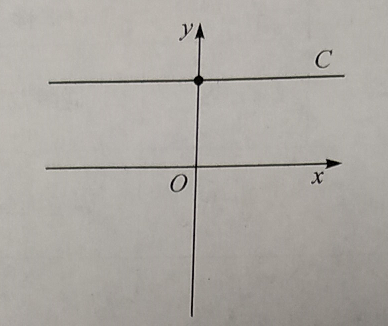2 幂函数 y=xμ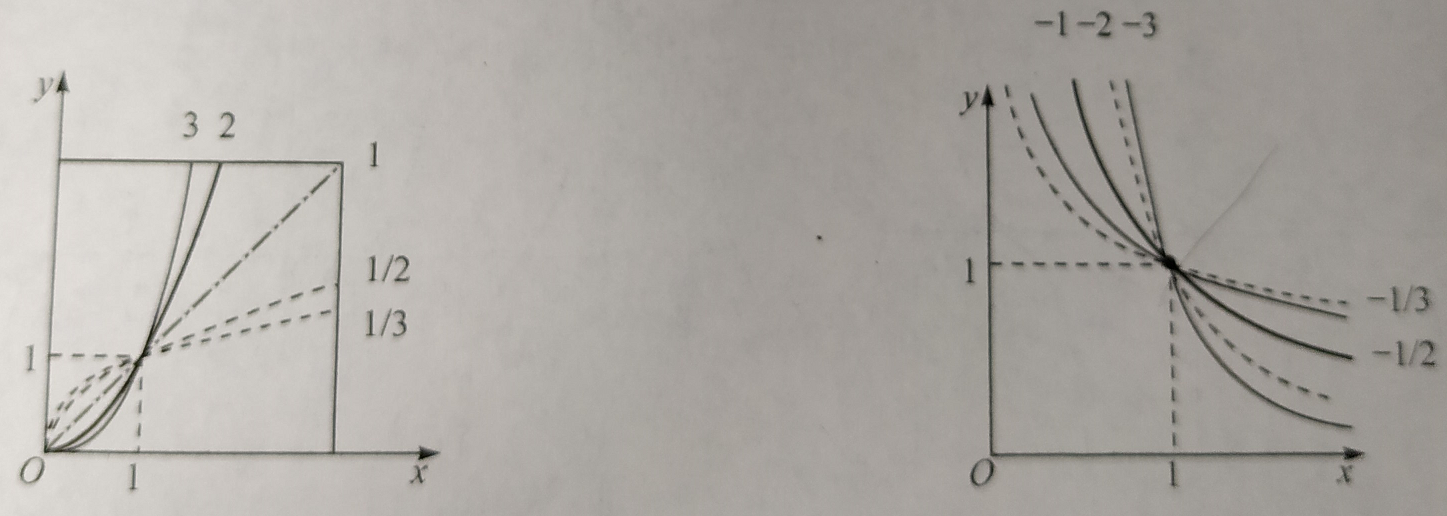3指数函数 y=ax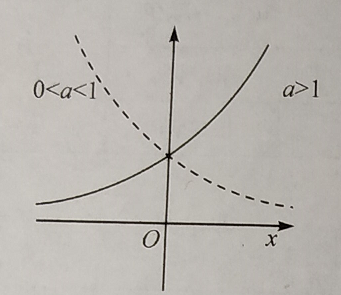4 对数函数 y=logax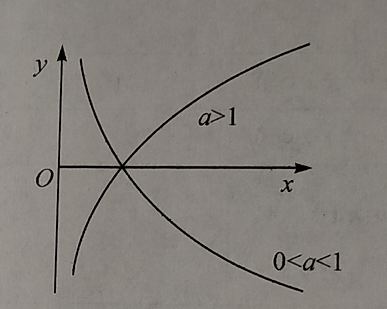5 正弦函数 y=sin(x)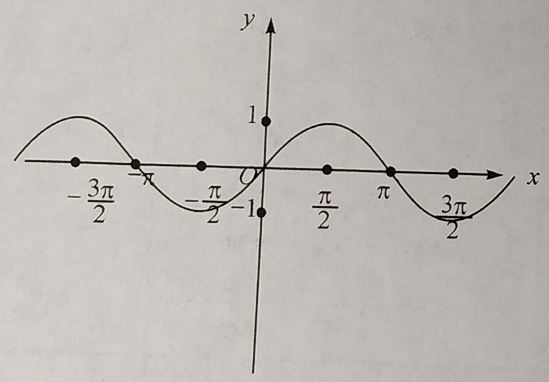6 余弦函数 y=cos(x)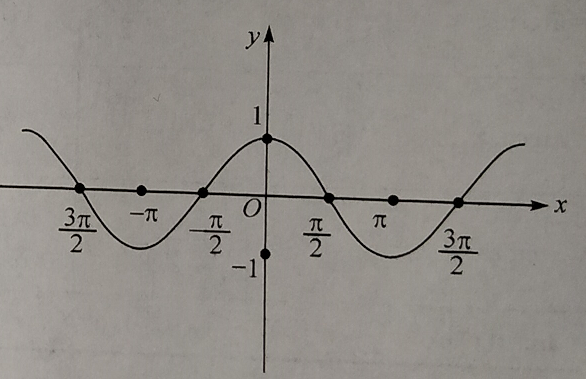7 正切函数 y=tan(x)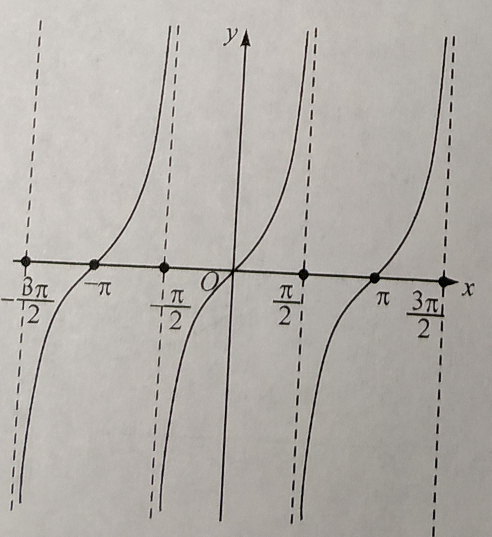8 余切函数 y=cot(x)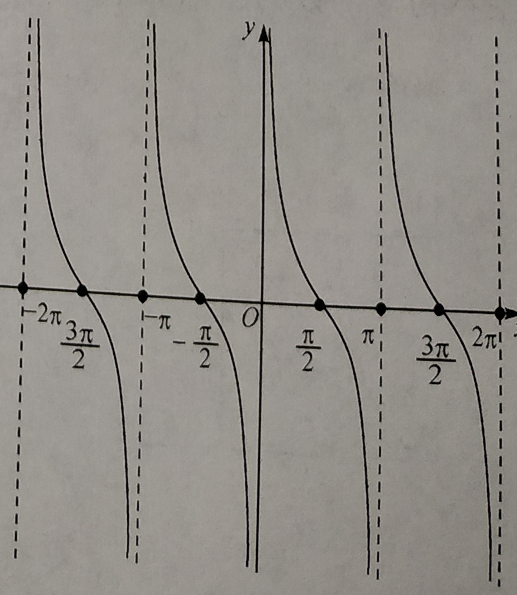9 反正弦函数 y=arcsin(x)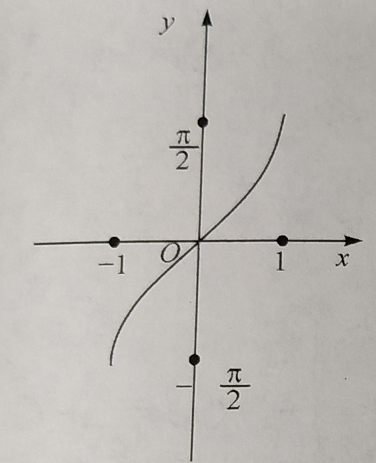10 反余弦函数 y=arccos(x)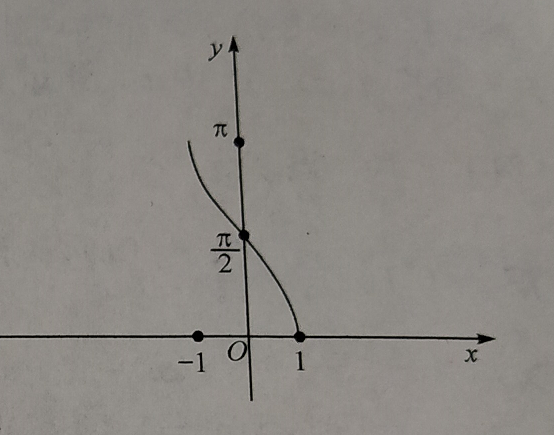11 反正切函数 y=arctan(x）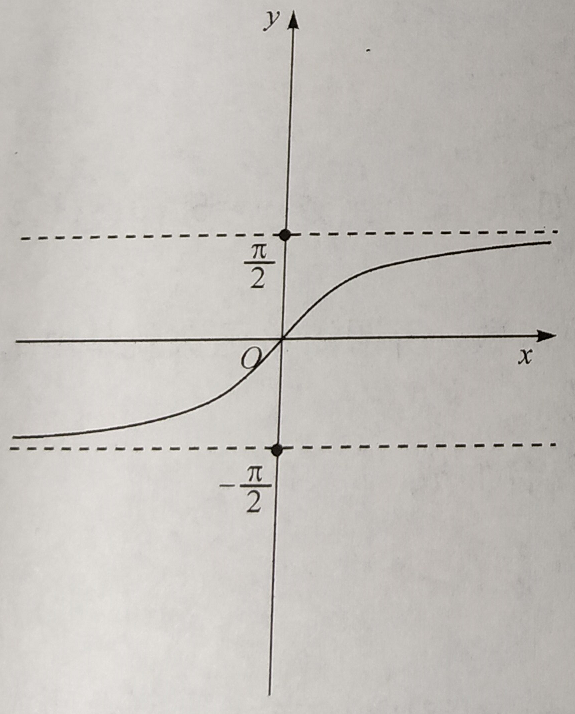12 反余切函数 y=arccot(x)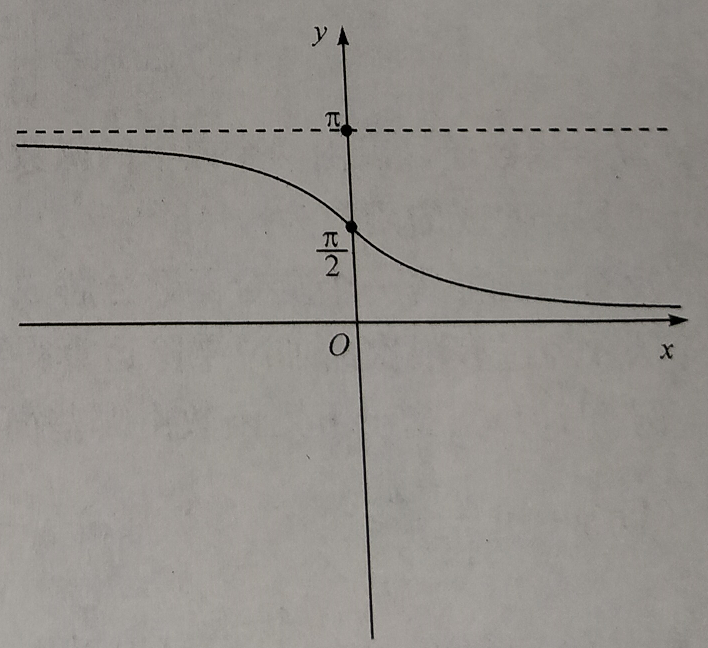函数的基本性质
1 有界性 2 单调性 设函数f(x)在D上有定义，如果对于D中任意两个数x1, x2, 当x1 < x2时，总有f(x1)<f(x2) (或 f(x1)>f(x2)), 则称f(x)在D上单调增加（或单调减少） 单调增加或单调减少的函数统称为单调函数。 3 奇偶性 设y=f(x), x∈D, 其中D关于原点对称，如果对于任意x∈D,总有 f(-x) = -f(x) (或 f(-x) = f(x))， 则称f(x)为奇函数（或偶函数）。 法则：两个偶函数的和是偶函数，两个奇函数的和是奇函数，两个偶函数的乘积是偶函数，两个奇函数的乘积也是偶函数，偶函数与奇函数的乘积是奇函数，偶函数与奇函数的和既不是偶函数也不是奇函数 4 周期性
反函数
展开全文函数曲线
•文档
•技术
• 初等函数   基本初等函数分为如下5类： 幂函数，如y=xμy = x^{\mu}y=xμ。...  初等函数是由基本初等函数经过有限次的四则运算复合运算所得到的函数。 二次函数   一般地，把形如y=ax2+bx+c  (a≠0)y =


文章目录
初等函数二次函数指数函数幂函数对数函数反函数分段函数

初等函数
基本初等函数分为如下5类：
幂函数，如

y

=

x

μ

y = x^{\mu}

。指数函数，如

y

=

a

x

y = a^x

。对数函数，如

y

=

l

o

g

a

x

y = log_{a}x

。三角函数，如

y

=

s

i

n

x

y = sin \; x

。反三角函数，如

y

=

a

r

c

s

i

n

x

y = arcsin \; x

。
初等函数是由基本初等函数经过有限次的四则运算和复合运算所得到的函数。
二次函数
一般地，把形如

y

=

a

x

2

+

b

x

+

c

(

a

≠

0

)

y = ax^2 + bx + c \; (a \neq 0)

的函数叫做二次函数，其中

a

a

称为二次项系数，

b

b

为一次项系数，

c

c

为常数项。x为自变量，y为因变量。   二次函数的图像形如物体抛射时所经过的路径，我们把它叫做抛物线。对称轴与抛物线的交点叫做抛物线的顶点。顶点是抛物线的最高点或最低点。   当

a

>

0

a > 0

时，抛物线

y

=

a

x

2

y = ax^2

的对称轴是y轴，顶点是原点，开口向上，顶点是抛物线的最低点，

a

a

越大，抛物线的开口越小。   当

a

<

0

a < 0

时，抛物线

y

=

a

x

2

y = ax^2

的对称轴是y轴，顶点是原点，开口向下，顶点是抛物线的最高点，

∣

a

∣

\left | a \right |

越大，抛物线的开口越小。   抛物线

y

=

a

x

2

+

k

y = ax^2 + k

的图像可由

y

=

a

x

2

y = ax^2

图像向上或向下平移得到：当

k

>

0

k > 0

，向上平移；当

k

<

0

k < 0

，向下平移。   抛物线

y

=

a

x

2

+

k

y = ax^2 + k

的性质：
当

a

>

0

a > 0

时，开口向上；当

a

<

0

a < 0

时，开口向下。对称轴是y轴。顶点坐标是

(

0

,

k

)

(0, \; k)

。

∣

a

∣

\left | a \right |

越大，开口越小。
抛物线

y

=

a

(

x

−

h

)

2

y = a(x - h)^2

的性质：
当

a

>

0

a > 0

时，开口向上；当

a

<

0

a < 0

时，开口向下。对称轴是

x

=

h

x = h

。顶点坐标是

(

h

,

0

)

(h, \; 0)

。

∣

a

∣

\left | a \right |

越大，开口越小。
抛物线左右移动的原则是左加右减：
把抛物线

y

=

−

1

2

x

2

\displaystyle{y = -\frac{1}{2}x^2}

向左平移1个单位，就得到抛物线

y

=

−

1

2

(

x

+

1

)

2

\displaystyle{y = -\frac{1}{2}(x + 1)^2}

。把抛物线

y

=

−

1

2

x

2

\displaystyle{y = -\frac{1}{2}x^2}

向右平移1个单位，就得到抛物线

y

=

−

1

2

(

x

−

1

)

2

\displaystyle{y = -\frac{1}{2}(x - 1)^2}

。
抛物线

y

=

a

(

x

−

h

)

2

+

k

y = a(x - h)^2 + k

的性质：
当

a

>

0

a > 0

时，开口向上；当

a

<

0

a < 0

时，开口向下。对称轴是

x

=

h

x = h

。顶点坐标是

(

h

,

k

)

(h, \; k)

。

∣

a

∣

\left | a \right |

越大，开口越小。
把函数

y

=

a

x

2

+

b

x

+

c

y = ax^2 + bx + c

通过配方法变成顶点式

y

=

a

x

2

+

b

x

+

c

=

a

(

x

+

b

2

a

)

2

+

4

a

c

−

b

2

4

a

\displaystyle{y = ax^2 + bx + c = a(x + \frac{b}{2a})^2 + \frac{4ac - b^2}{4a}}

，其中对称轴是

x

=

−

b

2

a

\displaystyle{x = -\frac{b}{2a}}

，顶点是

(

−

b

2

a

,

4

a

c

−

b

2

4

a

)

\displaystyle{(-\frac{b}{2a}, \; \frac{4ac - b^2}{4a})}

。   二次函数

y

=

a

x

2

+

b

x

+

c

y = ax^2 + bx + c

的图像与x轴交点的个数：
如果

b

2

−

4

a

c

>

0

b^2 - 4ac > 0

，则有2个交点。如果

b

2

−

4

a

c

=

0

b^2 - 4ac = 0

，则有1个交点。如果

b

2

−

4

a

c

<

0

b^2 - 4ac < 0

，则没有交点。
指数函数
一般地，函数

y

=

a

x

(

a

>

0

且

a

≠

1

)

y = a^x \; (a > 0且a \neq 1)

叫做指数函数，函数的定义域是R。指数函数都经过点

(

0

,

1

)

(0, \; 1)

。   当

a

>

1

a > 1

时，指数函数都是增函数；当

0

<

a

<

1

0 < a < 1

时，指数函数都是减函数。
幂函数
一般地，函数

y

=

x

α

y = x^\alpha

称为幂函数，其中x是自变量，

α

\alpha

是常量。   当

α

>

0

\alpha > 0

时，幂函数

y

=

x

α

y = x^\alpha

有下列性质：
图像都经过点

(

1

,

1

)

(1, \; 1)

和

(

0

,

0

)

(0, \; 0)

。函数的图像在区间

[

0

,

+

∞

)

[0, \; +\infty)

上是增函数。
当

α

<

0

\alpha < 0

时，幂函数

y

=

x

α

y = x^\alpha

有下列性质：
图像都经过点

(

1

,

1

)

(1, \; 1)

。函数的图像在区间

(

0

,

+

∞

)

(0, \; +\infty)

上是减函数。
当

α

\alpha

为奇数时，幂函数是奇函数；当

α

\alpha

为偶数时，幂函数是偶函数。
对数函数
一般地，函数

y

=

l

o

g

a

x

(

a

>

0

且

a

≠

1

)

y = log_{a}x \; (a > 0且a \neq 1)

叫做对数函数，其中x是自变量，函数的定义域是

(

0

,

+

∞

)

(0, \; +\infty)

，值域为R。指数函数过点

(

0

,

1

)

(0, \; 1)

。   当

a

>

1

a > 1

时，具有如下性质：
当

0

<

x

<

1

0 < x < 1

时，

y

<

0

y < 0

；当

x

>

1

x > 1

时，

y

>

0

y > 0

。在

(

0

,

+

∞

)

(0, \; +\infty)

上是增函数。
当

0

<

a

<

1

0 < a < 1

时，具有如下性质：
当

0

<

x

<

1

0 < x < 1

时，

y

>

0

y > 0

；当

x

>

1

x > 1

时，

y

<

0

y < 0

。在

(

0

,

+

∞

)

(0, \; +\infty)

上是减函数。
反函数
设函数

y

=

f

(

x

)

y = f(x)

的定义域是D，值域是f(D)，如果对于值域f(D)中的每一个y，在D中有且只有一个x使得

g

(

y

)

=

x

g(y) = x

，则按此对应法则得到了一个定义在f(D)上的函数，并把该函数称为函数

y

=

f

(

x

)

y = f(x)

的反函数，记为

x

=

f

−

1

(

y

)

,

y

∈

f

(

D

)

x = f^{-1}(y), \; y \in f(D)

。   函数

f

f

的定义域D和值域f(D)恰好就是反函数

f

−

1

f^{-1}

的值域和定义域，并且

f

−

1

f^{-1}

的反函数就是

f

f

，也就是说，函数

f

f

和

f

−

1

f^{-1}

互为反函数。   相对于反函数

y

=

f

−

1

(

x

)

y = f^{-1}(x)

来说，原来的函数

y

=

f

(

x

)

y = f(x)

称为直接函数。反函数和直接函数的图像关于直线

y

=

x

y = x

对称。
分段函数
分段函数：对于自变量x的不同的取值范围，有着不同的解析式的函数，例如

f

(

x

)

=

{

1

0

x

x

≤

1

x

x

>

1

f(x) = \left\{\begin{matrix} 10^x & x \le 1 \\ x & x > 1 \end{matrix}\right.

。它是一个函数，而不是几个函数。   分段函数的定义域是各段函数定义域的并集，值域也是各段函数值域的并集。
展开全文数学
•文档
• 基本初等函数分为幂函数、指数函数、对数函数、三角函数、反三角函数。 幂函数： 指数函数： 对数函数： 三角函数： 正弦函数： 余弦函数： 正切函数： 余切函数： 正割函数： 余割函数： 其它：正矢函数、...
基本初等函数分为幂函数、指数函数、对数函数、三角函数、反三角函数。 初等函数是由幂函数（power function）、指数函数（exponential function）、对数函数（logarithmic function）、三角函数（trigonometric function）、反三角函数（inverse trigonometric function）与常数经过有限次的有理运算（加、减、乘、除、有理数次乘方、有理数次开方）及有限次函数复合所产生，并且能用一个解析式表示的函数。
幂函数：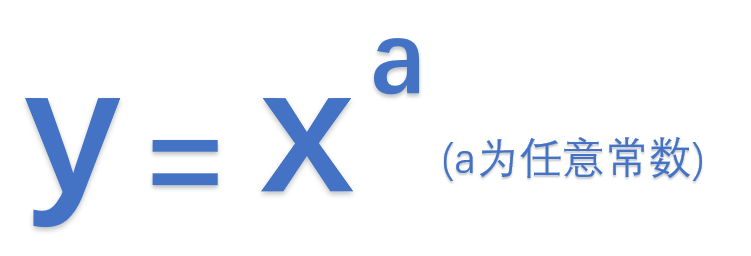指数函数：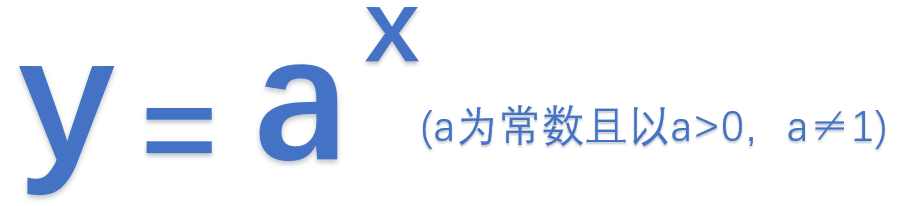对数函数：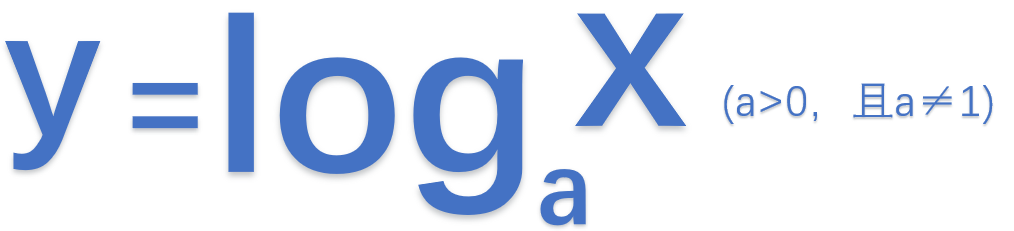三角函数：
正弦函数：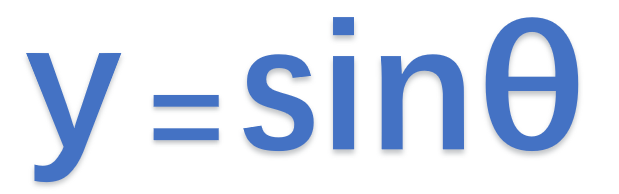余弦函数：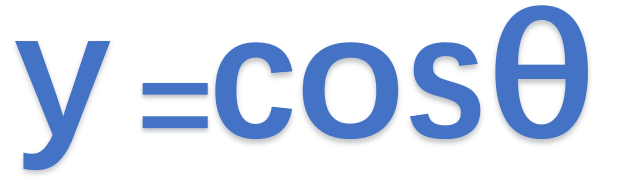正切函数：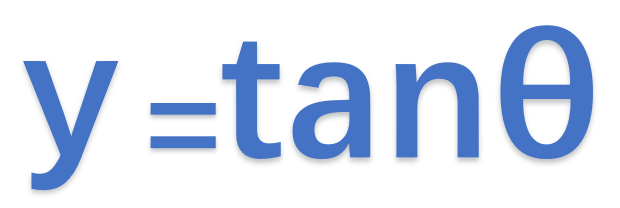余切函数：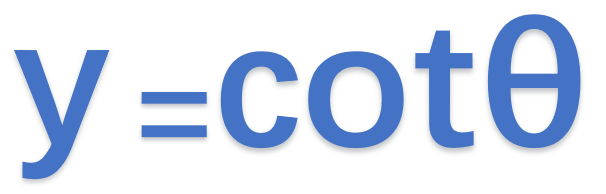正割函数：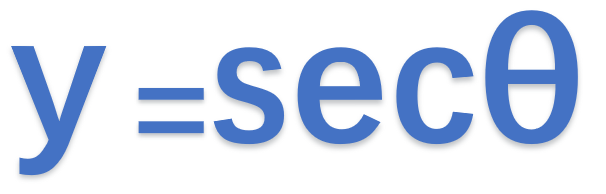余割函数：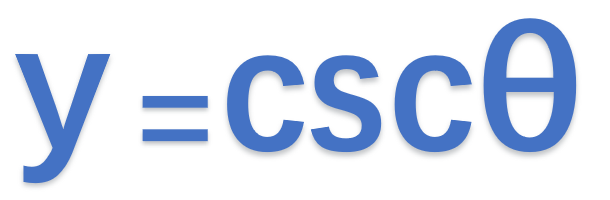其它：正矢函数、余矢函数、半正矢函数、半余矢函数、外正割函数、外余割函数。
反三角函数：
反正弦函数：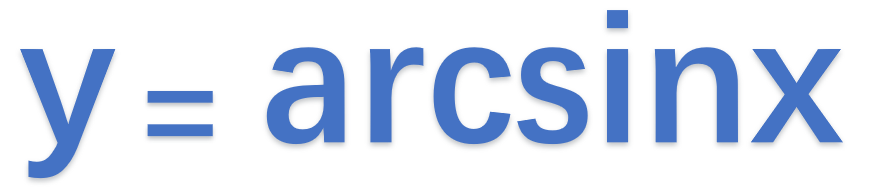反余弦函数：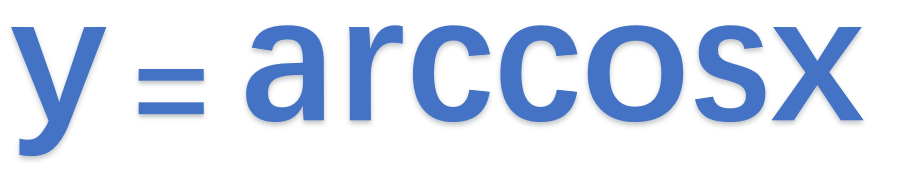反正切函数：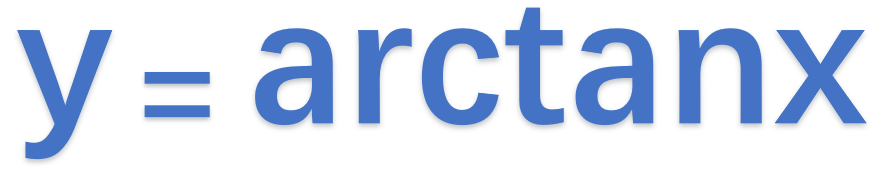反余切函数：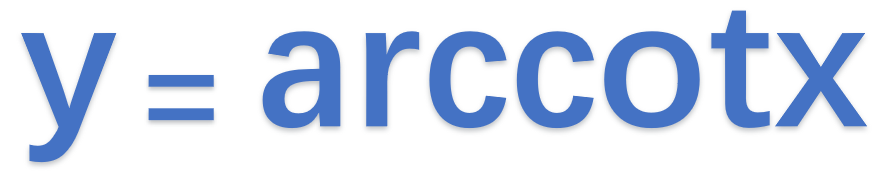反正割函数：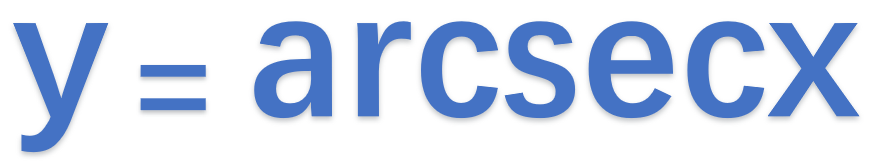反余割函数：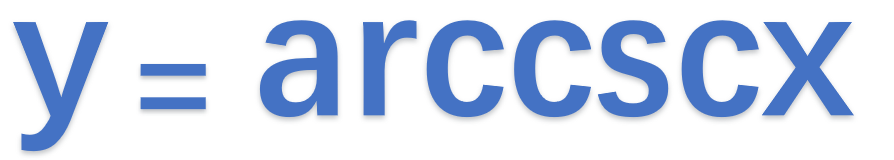由基本初等函数经过有限次四则运算、复合运算构成的有统一表达式的函数叫做初等函数。 初等函数有多项式函数和有理函数等。 通过对多项式进行代数运算（四则运算和开方运算）所得到的函数称为代数函数，非代数函数称为超越函数（比如三角函数、反三角函数、指数函数、对数函数）。
双曲函数
双曲正弦函数、双曲余弦函数、双曲正切函数、双曲余切函数. 更多：百度百科链接：https://baike.baidu.com/item/%E5%8F%8C%E6%9B%B2%E5%87%BD%E6%95%B0/8704306?fr=aladdin 双曲正弦：双曲余弦：双曲正切：双曲余切：双曲正割：双曲余割：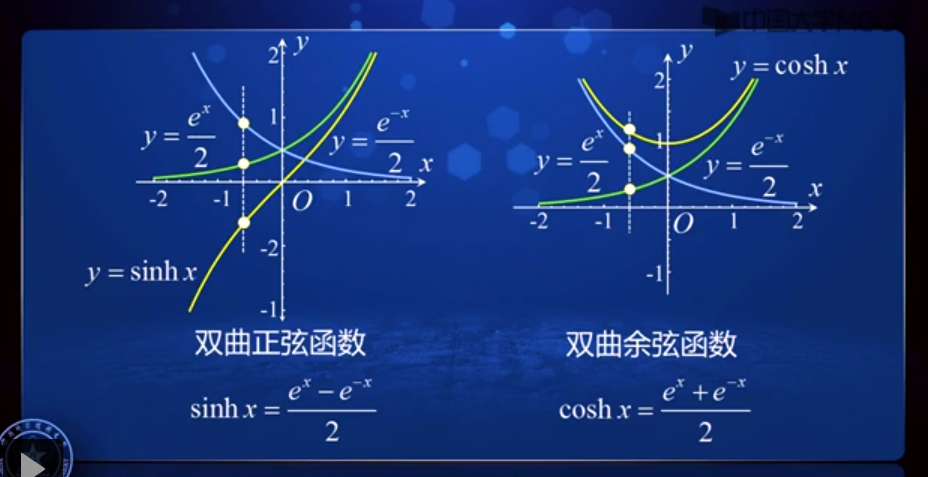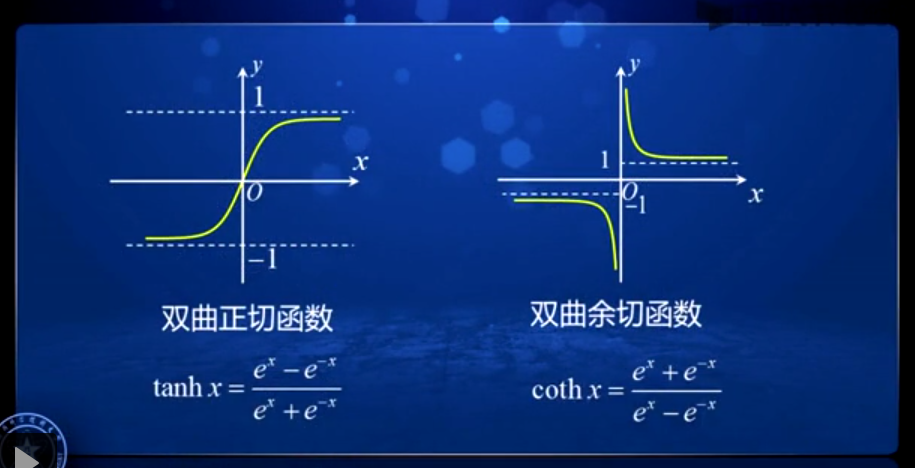公式： sh(x+y) = sh x·ch y + ch x·sh y ch(x+y) = ch x·ch y + sh x·sh y (ch x)2 - (sh x)2 = 1
反双曲正弦 y = arsh x = ln(x + √(x2+1)) x∈(-∞,+∞)奇函数,定义域内单调增反双曲余弦 y = arch x = ln(x + √(x2-1)) x∈[0,+∞),定义域内单调增反双曲正切 y = arth x = (1/2)ln((1+x)/(1-x)) x∈(-1,1)奇函数,定义域内单调增tip：在线的matlab工具 https://octave-online.net/
展开全文• 辽宁省沈阳市2017_2018学年高中数学暑假作业集合函数基本初等函数2函数的基本概念
• 基本初等函数图像 极限判断 常用公式 不好 赔你 双倍分
• Matlab基本初等函数大全 方便查阅，适合基础学习者使用。 链接中是完整版 完整版初等函数大全 1、特殊变量与常数 ans 计算结果的变量名 computer 确定运行的计算机 eps 浮点相对精度 Inf 无穷大 I 虚数单位 input...
Matlab基本初等函数大全 方便查阅，适合基础学习者使用。 链接中是完整版 完整版初等函数大全 1、特殊变量与常数 ans 计算结果的变量名 computer 确定运行的计算机 eps 浮点相对精度 Inf 无穷大 I 虚数单位 inputname 输入参数名 NaN 非数 nargin 输入参数个数 nargout 输出参数的数目 pi 圆周率 asin,asinh 反正弦，反双曲正弦 atan,atanh 反正切，双曲正切 tangent 正切 atan2 四象限反正切
varargout 实际返回的参量 操作符与特殊字符
加 - 减
矩阵乘法 .* 数组乘（对应元素相乘） ^ 矩阵幂 .^ 数组幂（各个元素求幂） \ 左除或反斜杠 / 右除或斜面杠 ./ 数组除（对应元素除） kron Kronecker张量积 : 冒号 () 圆括 [] 方括 . 小数点
展开全文MATLAB
• 其中,关于基本初等函数的保密计算是所有云计算的基础核心。提出了所有基本初等函数的保密云计算服务协议,其基本思想是将原始数据做变换后,把计算复杂部分发给云平台,通过云计算将结果反馈给接受服务方,从而保密地...
• 基本初等函数图像 极限判断 常用公式 不好 赔你 双倍分 基本初等函数图像 极限判断 常用公式 不好 赔你 双倍分
• 初等函数是由常数和基本初等函数，通过四则运算或者复合运算得到的函数。在计算初等函数的导数时，通常会涉及到基本初等函数的导数计算，需要牢记。 常见的基本初等函数的导数公式： ...
• 2018年高考数学第二章函数概念与基本初等函数专题5函数基本性质考场高招大全
•文档
• 第二篇函数与基本初等函数Ⅰ第5讲对数与对数函数.pdf技术
• 学习第二篇函数与基本初等函数第5讲对数与对数函数.pdf技术
•文档
• 由常数和基本初等函数经过有限次的四则运算和有限次的函数复合步骤所构成并可用于一个解析式表示的函数，称为初等函数，例如: (幂函数复合),(三角函数和幂函数复合）(三角函数和幂函数复合）. 应用上常常遇到以为...
• 欢迎点击「算法与编程之美」↑关注我们！本文首发于微信公众号："算法与编程之美"，欢迎关注，及时了解更多此系列博客。在上一篇文章数学分析将基本初等函数归为六类：幂函数、指数......